# SAT Math Multiple Choice Question 835: Answer and Explanation

### Test Information

Question: 835

10. A bike messenger delivers a package from the courthouse to a law firm downtown, travelingat an average speed of 24 miles per hour. On the way back, the bike messenger getsstuck in traffic, which reduces his average speed for the return trip by 12.5 percent.What was the bike messenger's average speed, in miles per hour, for the round trip?

• A. 22
• B. 22.1
• C. 22.4
• D. 22.5

Explanation:

C

Difficulty: Medium

Category: Problem Solving and Data Analysis / Rates, Ratios, Proportions, and Percentages

Strategic Advice: In average speed problems, never take the average of the two average speeds. Instead, use the formula: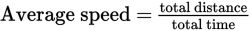Getting to the Answer: The bike messenger's average speed in one direction is 24 mph. On the way back, it's reduced by 12.5%, or 0.125 x 24 =3 or mph, for an average speed of 21 mph. The answer is NOT the average of these two speeds, 22.5, because that fails to account for the fact that the bike messenger spent more time traveling at the slower speed. Instead, use the average speed formula to find the answer.

You're not given the distance to the law firm, so pick a convenient number to use in your calculations. A good number to pick is 168 because it's divisible by both 21 and 24. Given that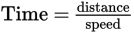, the time spent traveling from the courthouse is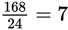hours, and the time spent traveling back is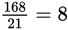hours, for a total time of 7 + 8 = 15 hours. The total distance there and back is 168 + 168 = 336 miles. You now have enough information to use the average speed formula: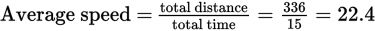, choice (C).